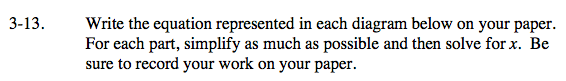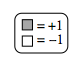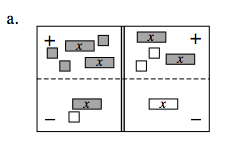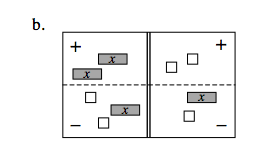### Home > CAAC > Chapter 3 > Lesson 3.1.2 > Problem3-13

3-13.Use the eTools below to help solve the problems.

Click the link at right for the full version of the eTool: AC 3-13a HW eTool

Remove zero pairs.

Combine like terms.

Remove an x-tile from both sides.

Divide by 2 on both sides.

2x + 3 + (−x) + 1 = 2x + (−2) + x

2x + (−x) + 3 + 1 = 2x + x + (−2)

x + 3 + 1 = 2x + x + (−2)

x + 4 = 3x − 2

4 = 2x − 2

6 = 2x

3 = xFollow the steps in part (a).

Click the link at right for the full version of the eTool: AC 3-13b HW eTool